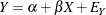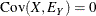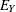### Simple Linear Regression

Consider fitting a linear equation to two observed variables, Y and X. Simple linear regression uses the following model form:The model makes the following assumption:The parametersandare the intercept and regression coefficient, respectively, andis an error term. If the values of X are fixed, the values ofare assumed to be independent and identically distributed realizations of a normally distributed random variable with mean zero and variance Var(). If X is a random variable, X andare assumed to have a bivariate normal distribution with zero correlation and variances Var(X) and Var(), respectively. Under either set of assumptions, the usual formulas hold for the estimates of the intercept and regression coefficient and their standard errors. (See Chapter 4: Introduction to Regression Procedures.)

In the REG procedure, you can fit a simple linear regression model with a MODEL statement that lists only the names of the manifest variables, as shown in the following statements:

proc reg;
model Y = X;
run;


You can also fit this model with PROC CALIS, but the syntax is different. You can specify the simple linear regression model in PROC CALIS by using the LINEQS modeling language, as shown in the following statements:

proc calis;
lineqs
Y = beta * X + Ey;
run;


LINEQS stands for LINear EQuationS. You invoke the LINEQS modeling language by using the LINEQS statement in PROC CALIS. In the LINEQS statement, you specify the linear equations of your model. The LINEQS statement syntax is similar to the mathematical equation that you would write for the model. An obvious difference between the LINEQS and the PROC REG model specification is that in LINEQS you can name the parameter involved (for example, beta) and you also specify the error term explicitly. The additional syntax required by the LINEQS statement seems to make the model specification more time-consuming and cumbersome. However, this inconvenience is minor and is offset by the modeling flexibility of the LINEQS modeling language (and of PROC CALIS, generally). As you proceed to more examples in this chapter, you will find the benefits of specifying parameter names for more complicated models with constraints. You will also find that specifying parameter names for unconstrained parameters is optional. Using parameter names in the current example is for the ease of reference in the current discussion.

You might wonder whether an intercept term is missing in the LINEQS statement and where you should put the intercept term if you want to specify it. The intercept term, which is considered as a mean structure parameter in the context of structural equation modeling, is usually omitted when statistical inferences can be drawn from analyzing the covariance structures alone. However, this does not mean that the regression equation has a default fixed-zero intercept in the LINEQS specification. Rather, it means only that the mean structures are saturated and are not estimated in the covariance structure model. Therefore, in the preceding LINEQS specification, the intercept termis implicitly assumed in the model. It is not of primary interest and is not estimated.

However, if you want to estimate the intercept, you can specify it in the LINEQS equations, as shown in the following specification:

proc calis;
lineqs
Y = alpha * Intercept + beta * X + Ey;
run;


In this LINEQS statement, alpha represents the intercept parameterand intercept represents an internal variable that has a fixed value of 1 for each observation. With this specification, an estimate ofis displayed in the PROC CALIS output results. However, estimation results for other parameters are the same as those from the specification without the intercept term. For this reason the intercept term is not specified in the examples of this section.